# Condenser

Cooling water flow calculation: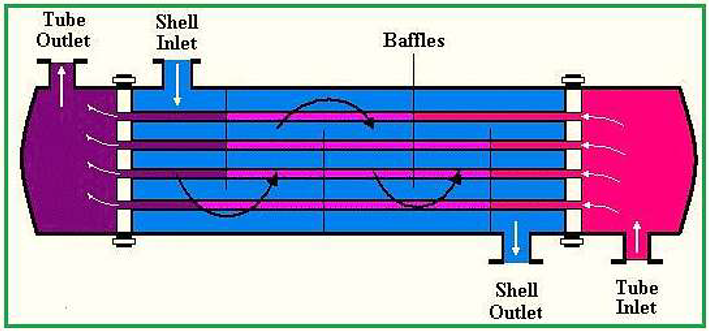Basic concept of specific heat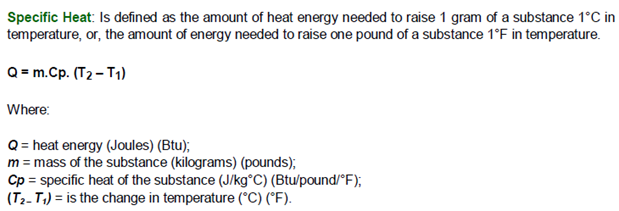Heat exchangers calculations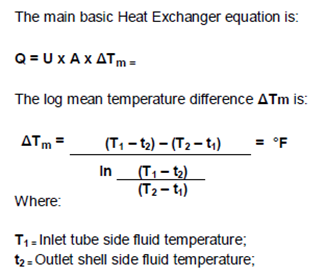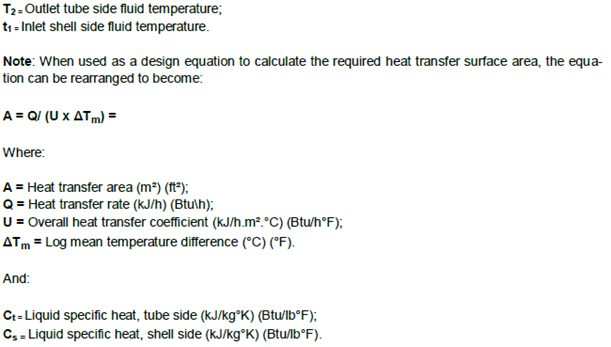Given

In our case, the heat exchanger already exists we want to calculate the flow of water needed. We're going to use this equation:

Q = M . Cp1. (Te - Ts) = m. Cp2. (ts - te)

with: M: flow of steam (kg/s)

m: flow of water (kg/s)

Cp1: Steam Specific heat (kj/kg.k)

Cp2: water Specific heat (kj/kg.k)

Te: Temperature of entering steam

Ts: Temperature of exiting steam

ts: Temperature of exiting water

te: Temperature of entering water

First, we have to find the specific heats: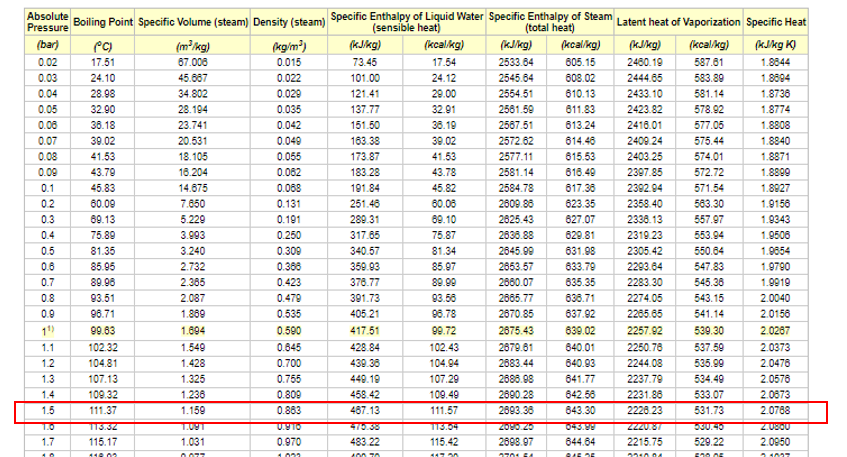at a pressure of 1.5 bar, the specific heat of steam is 2.0768 Kj/Kg.k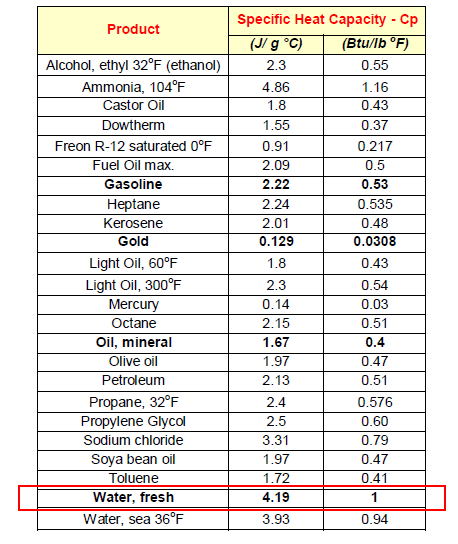the specific heat of fresh water is around 4.19 kj/kg.k

The temperature of cooling water used as the inlet is around 25 °C so te = 25 °C and will exit the condenser with a temperature around 50 °C.

The steam exiting the turbine and entering the condenser is around 120 °C so Te =120 °C, it should be cooled to over 90 °C so Ts = 90°C.

We also have the flow of steam around 50 g/s. We are now able to calculate the flow of water needed using the equation stated above.

Calculation and results

Q = M . Cp1. (Te - Ts) = m. Cp2. (ts - te)

50 g/s x 2.0768 kj/kg.k x (120-90) k = m x 4.19 kj/kg.k x (50-25) k

0.05 kg/s x 2.0768 kj/kg.k x (120-90) k = m x 4.19 kj/kg.k x (50-25) k

3.1152 Kj/s = 104.75 kj/kg x m

m = 0.029 kg/s = 30 g/s

multiplied by a safety factor of 1.5 we'll need around 45 g/s of fresh water. That means 162 liters/hour.